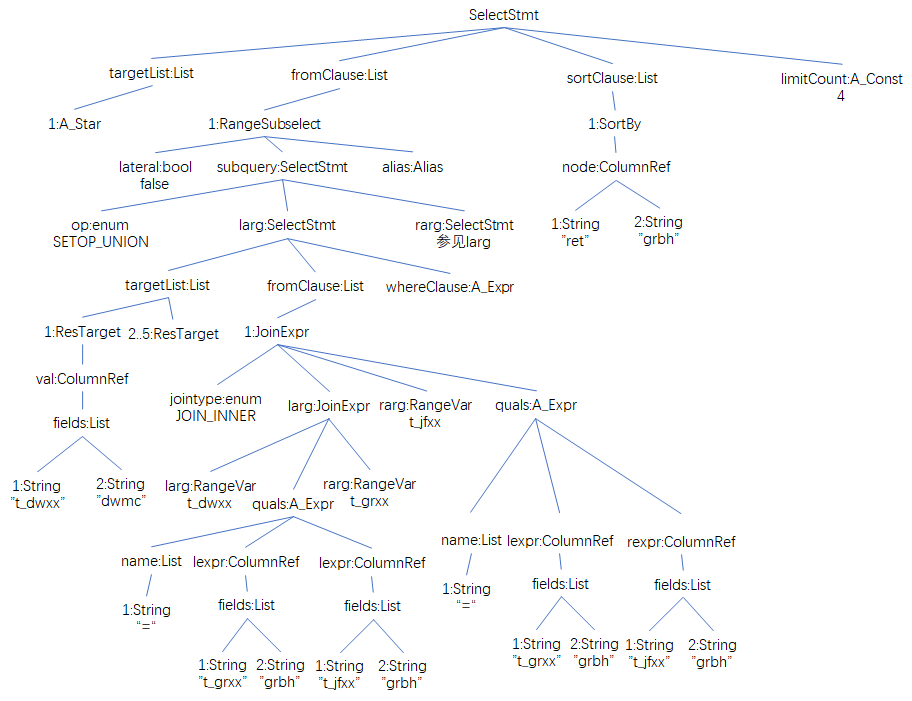# PostgreSQL 源码解读（19）- 查询语句#4（ParseTree详解）

### 一、解析树结构

```testdb=# select * from (
testdb(# select t_dwxx.dwmc,t_grxx.grbh,t_grxx.xm,t_jfxx.ny,t_jfxx.je
testdb(# from t_dwxx inner join t_grxx on t_dwxx.dwbh = t_grxx.dwbh
testdb(# inner join t_jfxx on t_grxx.grbh = t_jfxx.grbh
testdb(# where t_dwxx.dwbh IN ('1001')
testdb(# union all
testdb(# select t_dwxx.dwmc,t_grxx.grbh,t_grxx.xm,t_jfxx.ny,t_jfxx.je
testdb(# from t_dwxx inner join t_grxx on t_dwxx.dwbh = t_grxx.dwbh
testdb(# inner join t_jfxx on t_grxx.grbh = t_jfxx.grbh
testdb(# where t_dwxx.dwbh IN ('1002')
testdb(# ) as ret
testdb-# order by ret.grbh
testdb-# limit 4;
```

```(gdb) b exec_simple_query
Breakpoint 1 at 0x84cad8: file postgres.c, line 893.
(gdb) c
Continuing.
Breakpoint 1, exec_simple_query (
query_string=0x1641ef0 "select * from (\nselect t_dwxx.dwmc,t_grxx.grbh,t_grxx.xm,t_jfxx.ny,t_jfxx.je\nfrom t_dwxx inner join t_grxx on t_dwxx.dwbh = t_grxx.dwbh\ninner join t_jfxx on t_grxx.grbh = t_jfxx.grbh\nwhere t_dwxx.dwbh"...) at postgres.c:893
944     parsetree_list = pg_parse_query(query_string);
(gdb)
947     if (check_log_statement(parsetree_list))
#解析树中只有一个元素
(gdb) p *parsetree_list
\$2 = {type = T_List, length = 1, head = 0x1667180, tail = 0x1667180}
\$3 = {type = T_RawStmt}
\$4 = {type = T_RawStmt, stmt = 0x1666df0, stmt_location = 0, stmt_len = 455}
\$5 = {type = T_SelectStmt}
(gdb) set \$stmt=(SelectStmt *)(((RawStmt *)(parsetree_list->head.data->ptr_value))->stmt) #设置临时变量
(gdb) p *\$stmt
\$6 = {type = T_SelectStmt, distinctClause = 0x0, intoClause = 0x0, targetList = 0x1642ba0,
fromClause = 0x1666dc0, whereClause = 0x0, groupClause = 0x0, havingClause = 0x0, windowClause = 0x0,
valuesLists = 0x0, sortClause = 0x1667080, limitOffset = 0x0, limitCount = 0x16670b0, lockingClause = 0x0,
withClause = 0x0, op = SETOP_NONE, all = false, larg = 0x0, rarg = 0x0}
#op = SETOP_NONE,集合操作为NONE,只有一个SelectStmt,因此larg&rarg(类型为SelectStmt)为NULL
#------------------->targetList
(gdb) p *(\$stmt->targetList)
\$7 = {type = T_List, length = 1, head = 0x1642b80, tail = 0x1642b80}
\$8 = {type = T_ResTarget}
(gdb) p *\$restarget->val
\$9 = {type = T_ColumnRef}
(gdb) p *(ColumnRef *)\$restarget->val
\$10 = {type = T_ColumnRef, fields = 0x1642b00, location = 7}
(gdb) p *((ColumnRef *)\$restarget->val)->fields
\$11 = {type = T_List, length = 1, head = 0x1642ae0, tail = 0x1642ae0}
\$12 = {type = T_A_Star}
#实际类型是A_Star,即"*"
\$14 = {type = T_A_Star}
#------------------->fromClause
(gdb) p *\$stmt->fromClause
{type = T_List, length = 1, head = 0x1666da0, tail = 0x1666da0}
\$15 = {type = T_RangeSubselect} #实际类型是范围子查询RangeSubselect
(gdb) p *\$fromclause
\$16 = {type = T_RangeSubselect, lateral = false, subquery = 0x1666c18, alias = 0x1666d40}
(gdb) p *(\$fromclause->subquery) #subquery,子查询是SelectStmt类型的节点
\$17 = {type = T_SelectStmt}
(gdb) p *(\$fromclause->alias) #alias,别名,实际值是字符串ret
\$18 = {type = T_Alias, aliasname = 0x1666d28 "ret", colnames = 0x0}
#查看子查询结构体信息
#集合操作是SETOP_UNION,并∪操作,并操作的子查询在larg和rarg中
(gdb) p *(SelectStmt *)(\$fromclause->subquery)
\$20 = {type = T_SelectStmt, distinctClause = 0x0, intoClause = 0x0, targetList = 0x0, fromClause = 0x0,
whereClause = 0x0, groupClause = 0x0, havingClause = 0x0, windowClause = 0x0, valuesLists = 0x0,
sortClause = 0x0, limitOffset = 0x0, limitCount = 0x0, lockingClause = 0x0, withClause = 0x0,
op = SETOP_UNION, all = true, larg = 0x1665818, rarg = 0x1666b08}
#--->larg,左树节点
(gdb)  p *((SelectStmt *)(\$fromclause->subquery))->larg
\$21 = {type = T_SelectStmt, distinctClause = 0x0, intoClause = 0x0, targetList = 0x1642d50,
fromClause = 0x1643b38, whereClause = 0x1643d10, groupClause = 0x0, havingClause = 0x0, windowClause = 0x0,
valuesLists = 0x0, sortClause = 0x0, limitOffset = 0x0, limitCount = 0x0, lockingClause = 0x0,
withClause = 0x0, op = SETOP_NONE, all = false, larg = 0x0, rarg = 0x0}
(gdb) set \$subquerylarg=((SelectStmt *)(\$fromclause->subquery))->larg
#->targetList
(gdb) p *\$subquerylarg->targetList
\$25 = {type = T_List, length = 5, head = 0x1642d30, tail = 0x1643360}
\$26 = {type = T_ResTarget}
(gdb) p *\$subvar
{type = T_ResTarget, name = 0x0, indirection = 0x0, val = 0x1642c70, location = 23}
(gdb) p *(\$subvar->val)
\$29 = {type = T_ColumnRef}
(gdb) p *(ColumnRef *)(\$subvar->val)
\$30 = {type = T_ColumnRef, fields = 0x1642c40, location = 23}
\$33 = {type = T_String}
\$34 = {type = T_String, val = {ival = 23342032, str = 0x1642bd0 "t_dwxx"}}
(gdb) p *(Value *)(((ColumnRef *)(\$subvar->val))->fields)->tail.data->ptr_value
\$36 = {type = T_String, val = {ival = 23342056, str = 0x1642be8 "dwmc"}}
#->fromClause
(gdb) p *(\$subquerylarg->fromClause)
\$37 = {type = T_List, length = 1, head = 0x1643b18, tail = 0x1643b18}
\$38 = {type = T_JoinExpr}
\$39 = {type = T_JoinExpr, jointype = JOIN_INNER, isNatural = false, larg = 0x1643730, rarg = 0x1643798,
usingClause = 0x0, quals = 0x1643a08, alias = 0x0, rtindex = 0}
(gdb) p *(\$joinexpr->larg)
\$41 = {type = T_JoinExpr}
(gdb) p *(JoinExpr *)(\$joinexpr->larg)
\$42 = {type = T_JoinExpr, jointype = JOIN_INNER, isNatural = false, larg = 0x1643398, rarg = 0x1643400,
usingClause = 0x0, quals = 0x1643670, alias = 0x0, rtindex = 0}
(gdb) p *((JoinExpr *)(\$joinexpr->larg))->larg
\$43 = {type = T_RangeVar}
(gdb) p *(RangeVar *)((JoinExpr *)(\$joinexpr->larg))->larg
\$44 = {type = T_RangeVar, catalogname = 0x0, schemaname = 0x0, relname = 0x1643380 "t_dwxx", inh = true,
relpersistence = 112 'p', alias = 0x0, location = 82}
(gdb) p *((JoinExpr *)(\$joinexpr->larg))->rarg
\$45 = {type = T_RangeVar}
(gdb) p *(RangeVar *)((JoinExpr *)(\$joinexpr->larg))->rarg
\$46 = {type = T_RangeVar, catalogname = 0x0, schemaname = 0x0, relname = 0x16433e8 "t_grxx", inh = true,
relpersistence = 112 'p', alias = 0x0, location = 100}
(gdb) set \$expr=(A_Expr *)((JoinExpr *)(\$joinexpr->larg))->quals
{type = T_String, val = {ival = 11157870, str = 0xaa416e "="}}
{type = T_String, val = {ival = 23344208, str = 0x1643450 "t_dwxx"}}
(gdb) p *(Value *)(((ColumnRef *)\$expr->lexpr)->fields->tail->data.ptr_value)
{type = T_String, val = {ival = 23344232, str = 0x1643468 "dwbh"}}
{type = T_String, val = {ival = 23344480, str = 0x1643560 "t_grxx"}}
(gdb) p *(Value *)(((ColumnRef *)\$expr->rexpr)->fields->tail->data.ptr_value)
{type = T_String, val = {ival = 23344504, str = 0x1643578 "dwbh"}}
(gdb) p *(\$joinexpr->rarg)
\$47 = {type = T_RangeVar}
(gdb) p *(RangeVar *)(\$joinexpr->rarg)
\$48 = {type = T_RangeVar, catalogname = 0x0, schemaname = 0x0, relname = 0x1643780 "t_jfxx", inh = true,
relpersistence = 112 'p', alias = 0x0, location = 147}
(gdb) p *(\$joinexpr->quals)
\$49 = {type = T_A_Expr}
(gdb) p *(A_Expr *)(\$joinexpr->quals)
\$51 = {type = T_A_Expr, kind = AEXPR_OP, name = 0x1643a98, lexpr = 0x1643888, rexpr = 0x1643998,
location = 169}(gdb)
(gdb) p *((A_Expr *)(\$joinexpr->quals))->name
\$52 = {type = T_List, length = 1, head = 0x1643a78, tail = 0x1643a78}
(gdb) set \$expr=(A_Expr *)(\$joinexpr->quals)
\$53 = {type = T_String}
\$54 = {type = T_String, val = {ival = 11157870, str = 0xaa416e "="}}
(gdb) p *(\$expr->lexpr)
\$55 = {type = T_ColumnRef}
\$56 = {type = T_String, val = {ival = 23345128, str = 0x16437e8 "t_grxx"}}
(gdb) p *(Value *)(((ColumnRef *)\$expr->lexpr)->fields->tail->data.ptr_value)
\$57 = {type = T_String, val = {ival = 23345152, str = 0x1643800 "grbh"}}
(gdb) p *(\$expr->rexpr)
\$58 = {type = T_ColumnRef}
\$59 = {type = T_String, val = {ival = 23345400, str = 0x16438f8 "t_jfxx"}}
(gdb) p *(Value *)(((ColumnRef *)\$expr->rexpr)->fields->tail->data.ptr_value)
\$60 = {type = T_String, val = {ival = 23345424, str = 0x1643910 "grbh"}}
#--->rarg,右树节点
#参见larg
#------------------->sortClause
(gdb) p *\$stmt->sortClause
\$67 = {type = T_List, length = 1, head = 0x1667060, tail = 0x1667060}
\$69 = {type = T_SortBy}
\$70 = {type = T_SortBy, node = 0x1666fa0, sortby_dir = SORTBY_DEFAULT, sortby_nulls = SORTBY_NULLS_DEFAULT,
useOp = 0x0, location = -1}
\$71 = {type = T_SortBy, node = 0x1666fa0, sortby_dir = SORTBY_DEFAULT, sortby_nulls = SORTBY_NULLS_DEFAULT,
useOp = 0x0, location = -1}
(gdb) p *\$sortby->node
\$72 = {type = T_ColumnRef}
\$74 = {type = T_String, val = {ival = 23490304, str = 0x1666f00 "ret"}}
\$75 = {type = T_String, val = {ival = 23490328, str = 0x1666f18 "grbh"}}
#------------------->limitCount
(gdb)  p *\$stmt->limitCount
\$78 = {type = T_A_Const}
(gdb)  p *(A_Const *)\$stmt->limitCount
\$79 = {type = T_A_Const, val = {type = T_Integer, val = {ival = 4, str = 0x4 <Address 0x4 out of bounds>}},
location = 454}
```### 二、数据结构

1、SelectStmt

```/* ----------------------
*      Select Statement
*
* A "simple" SELECT is represented in the output of gram.y by a single
* SelectStmt node; so is a VALUES construct.  A query containing set
* operators (UNION, INTERSECT, EXCEPT) is represented by a tree of SelectStmt
* nodes, in which the leaf nodes are component SELECTs and the internal nodes
* represent UNION, INTERSECT, or EXCEPT operators.  Using the same node
* type for both leaf and internal nodes allows gram.y to stick ORDER BY,
* LIMIT, etc, clause values into a SELECT statement without worrying
* whether it is a simple or compound SELECT.
* ----------------------
*/
typedef enum SetOperation
{
SETOP_NONE = 0,
SETOP_UNION,
SETOP_INTERSECT,
SETOP_EXCEPT
} SetOperation;

typedef struct SelectStmt
{
NodeTag     type;

/*
* These fields are used only in "leaf" SelectStmts.
*/
List       *distinctClause; /* NULL, list of DISTINCT ON exprs, or
* lcons(NIL,NIL) for all (SELECT DISTINCT) */
IntoClause *intoClause;     /* target for SELECT INTO */
List       *targetList;     /* the target list (of ResTarget) */
List       *fromClause;     /* the FROM clause */
Node       *whereClause;    /* WHERE qualification */
List       *groupClause;    /* GROUP BY clauses */
Node       *havingClause;   /* HAVING conditional-expression */
List       *windowClause;   /* WINDOW window_name AS (...), ... */

/*
* In a "leaf" node representing a VALUES list, the above fields are all
* null, and instead this field is set.  Note that the elements of the
* sublists are just expressions, without ResTarget decoration. Also note
* that a list element can be DEFAULT (represented as a SetToDefault
* node), regardless of the context of the VALUES list. It's up to parse
* analysis to reject that where not valid.
*/
List       *valuesLists;    /* untransformed list of expression lists */

/*
* These fields are used in both "leaf" SelectStmts and upper-level
* SelectStmts.
*/
List       *sortClause;     /* sort clause (a list of SortBy's) */
Node       *limitOffset;    /* # of result tuples to skip */
Node       *limitCount;     /* # of result tuples to return */
List       *lockingClause;  /* FOR UPDATE (list of LockingClause's) */
WithClause *withClause;     /* WITH clause */

/*
* These fields are used only in upper-level SelectStmts.
*/
SetOperation op;            /* type of set op */
bool        all;            /* ALL specified? */
struct SelectStmt *larg;    /* left child */
struct SelectStmt *rarg;    /* right child */
/* Eventually add fields for CORRESPONDING spec here */
} SelectStmt;
```

2、RangeSubselect

``` /*
* RangeSubselect - subquery appearing in a FROM clause
*/
typedef struct RangeSubselect
{
NodeTag     type;
bool        lateral;        /* does it have LATERAL prefix? */
Node       *subquery;       /* the untransformed sub-select clause */
Alias      *alias;          /* table alias & optional column aliases */
} RangeSubselect;
```

3、Alias

```/*
* Alias -
*    specifies an alias for a range variable; the alias might also
*    specify renaming of columns within the table.
*
* Note: colnames is a list of Value nodes (always strings).  In Alias structs
* associated with RTEs, there may be entries corresponding to dropped
* columns; these are normally empty strings ("").  See parsenodes.h for info.
*/
typedef struct Alias
{
NodeTag     type;
char       *aliasname;      /* aliased rel name (never qualified) */
List       *colnames;       /* optional list of column aliases */
} Alias;
```

4、ResTarget

``` /*
* ResTarget -
*    result target (used in target list of pre-transformed parse trees)
*
* In a SELECT target list, 'name' is the column label from an
* 'AS ColumnLabel' clause, or NULL if there was none, and 'val' is the
* value expression itself.  The 'indirection' field is not used.
*
* INSERT uses ResTarget in its target-column-names list.  Here, 'name' is
* the name of the destination column, 'indirection' stores any subscripts
* attached to the destination, and 'val' is not used.
*
* In an UPDATE target list, 'name' is the name of the destination column,
* 'indirection' stores any subscripts attached to the destination, and
* 'val' is the expression to assign.
*
* See A_Indirection for more info about what can appear in 'indirection'.
*/
typedef struct ResTarget
{
NodeTag     type;
char       *name;           /* column name or NULL */
List       *indirection;    /* subscripts, field names, and '*', or NIL */
Node       *val;            /* the value expression to compute or assign */
int         location;       /* token location, or -1 if unknown */
} ResTarget;
```

5、FromExpr

``` /*----------
* FromExpr - represents a FROM ... WHERE ... construct
*
* This is both more flexible than a JoinExpr (it can have any number of
* children, including zero) and less so --- we don't need to deal with
* aliases and so on.  The output column set is implicitly just the union
* of the outputs of the children.
*----------
*/
typedef struct FromExpr
{
NodeTag     type;
List       *fromlist;       /* List of join subtrees */
Node       *quals;          /* qualifiers on join, if any */
} FromExpr;
```

6、JoinExpr

``` /*----------
* JoinExpr - for SQL JOIN expressions
*
* isNatural, usingClause, and quals are interdependent.  The user can write
* only one of NATURAL, USING(), or ON() (this is enforced by the grammar).
* If he writes NATURAL then parse analysis generates the equivalent USING()
* list, and from that fills in "quals" with the right equality comparisons.
* If he writes USING() then "quals" is filled with equality comparisons.
* If he writes ON() then only "quals" is set.  Note that NATURAL/USING
* are not equivalent to ON() since they also affect the output column list.
*
* alias is an Alias node representing the AS alias-clause attached to the
* join expression, or NULL if no clause.  NB: presence or absence of the
* alias has a critical impact on semantics, because a join with an alias
* restricts visibility of the tables/columns inside it.
*
* During parse analysis, an RTE is created for the Join, and its index
* is filled into rtindex.  This RTE is present mainly so that Vars can
* be created that refer to the outputs of the join.  The planner sometimes
* generates JoinExprs internally; these can have rtindex = 0 if there are
* no join alias variables referencing such joins.
*----------
*/
typedef struct JoinExpr
{
NodeTag     type;
JoinType    jointype;       /* type of join */
bool        isNatural;      /* Natural join? Will need to shape table */
Node       *larg;           /* left subtree */
Node       *rarg;           /* right subtree */
List       *usingClause;    /* USING clause, if any (list of String) */
Node       *quals;          /* qualifiers on join, if any */
Alias      *alias;          /* user-written alias clause, if any */
int         rtindex;        /* RT index assigned for join, or 0 */
} JoinExpr;
```

7、RangeVar

``` /*
* RangeVar - range variable, used in FROM clauses
*
* Also used to represent table names in utility statements; there, the alias
* field is not used, and inh tells whether to apply the operation
* recursively to child tables.  In some contexts it is also useful to carry
* a TEMP table indication here.
*/
typedef struct RangeVar
{
NodeTag     type;
char       *catalogname;    /* the catalog (database) name, or NULL */
char       *schemaname;     /* the schema name, or NULL */
char       *relname;        /* the relation/sequence name */
bool        inh;            /* expand rel by inheritance? recursively act
* on children? */
char        relpersistence; /* see RELPERSISTENCE_* in pg_class.h */
Alias      *alias;          /* table alias & optional column aliases */
int         location;       /* token location, or -1 if unknown */
} RangeVar;
```

8、ColumnRef

``` /*
* ColumnRef - specifies a reference to a column, or possibly a whole tuple
*
* The "fields" list must be nonempty.  It can contain string Value nodes
* (representing names) and A_Star nodes (representing occurrence of a '*').
* Currently, A_Star must appear only as the last list element --- the grammar
* is responsible for enforcing this!
*
* Note: any array subscripting or selection of fields from composite columns
* is represented by an A_Indirection node above the ColumnRef.  However,
* for simplicity in the normal case, initial field selection from a table
* name is represented within ColumnRef and not by adding A_Indirection.
*/
typedef struct ColumnRef
{
NodeTag     type;
List       *fields;         /* field names (Value strings) or A_Star */
int         location;       /* token location, or -1 if unknown */
} ColumnRef;
```

### 三、小结

1、解析树：通过跟踪分析源码，分析解析树Parsetree的结构；
2、其他数据结构：SelectSmt、RangeSubselect、JoinExpr等数据结构。

ITPUB数据库版块资深版主，对Oracle、PostgreSQL有深入研究。

• 博文量
1473
• 访问量
3906084